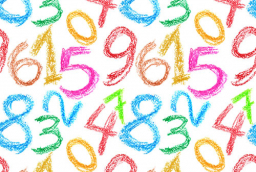# Divide 47913

I think the number. If I take six from it and divide the result by two, I get 6. What number do I think?

x =  18

### Step-by-step explanation:Did you find an error or inaccuracy? Feel free to write us. Thank you!

Tips for related online calculators
Do you have a linear equation or system of equations and looking for its solution? Or do you have a quadratic equation?